## Topic: Sorting

Free Printable Worksheets on Sorting Numbers and Words

## Sort the Numbers from Smallest to Largest (Set 1)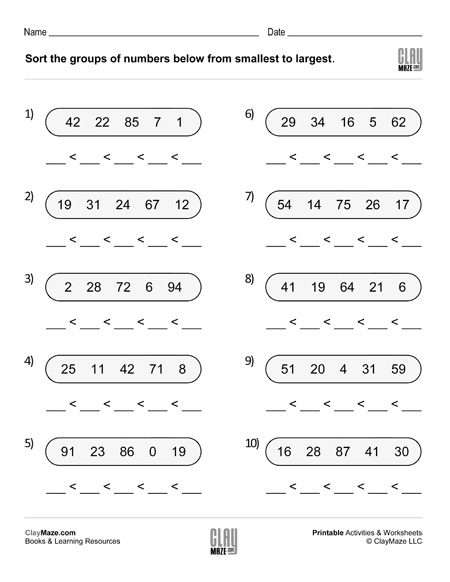This is an exercise in sorting numbers from least to greatest. There are 5 numbers in each group. – 10 problems...

## Sort the Numbers from Smallest to Largest (Set 2)Help your child develop sorting skills with this worksheet. Sort each group of five numbers. – 10 problems per page...

## Sort the Numbers from Smallest to Largest (Set 3)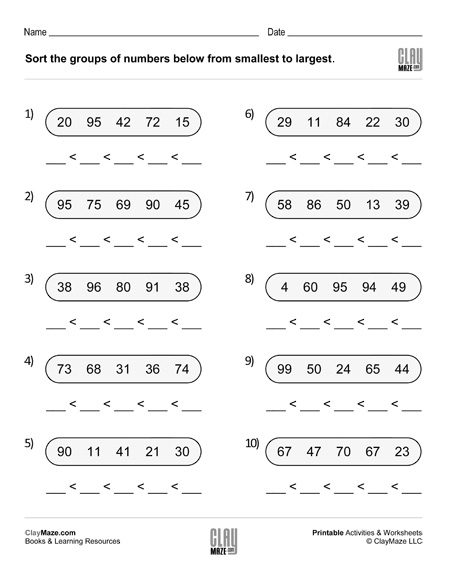Sort each group of 5 numbers from least to greatest. – 10 problems...

## Sort the Numbers from Smallest to Largest (Set 4)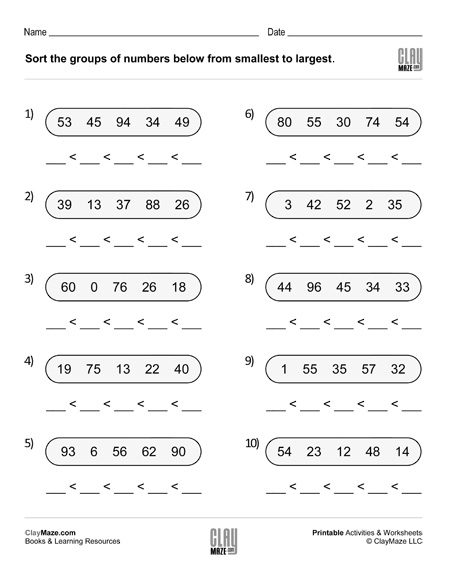Number sorting exercises – Sort each group of numbers from smallest to largest. – 20 problems...

## Fill in the Venn Diagrams (Set 1)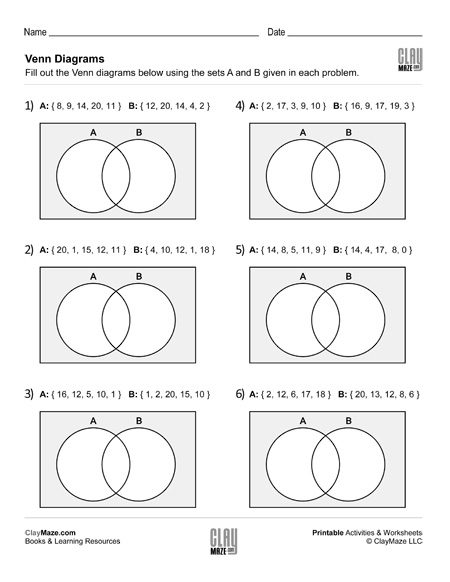Venn diagrams – Use the numbers sets to fill in the Venn diagrams. For more explanation of what a Venn diagram is and to see an example, click here.  ...

## Fill in the Venn Diagrams (Set 2)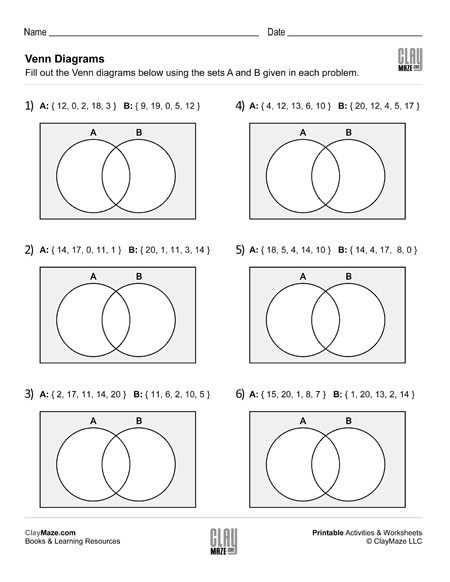Help your child develop an better understanding of logical relationships between  number sets. For more explanation of what a Venn diagram is and to see an example, click here....

## Fill in the Venn Diagrams (Set 3)Help your child develop an better understanding of number sets and how they are related as well as recording and reading data. For more explanation of what a Venn diagram is and to see an...

## Fill in the Venn Diagrams (Set 4)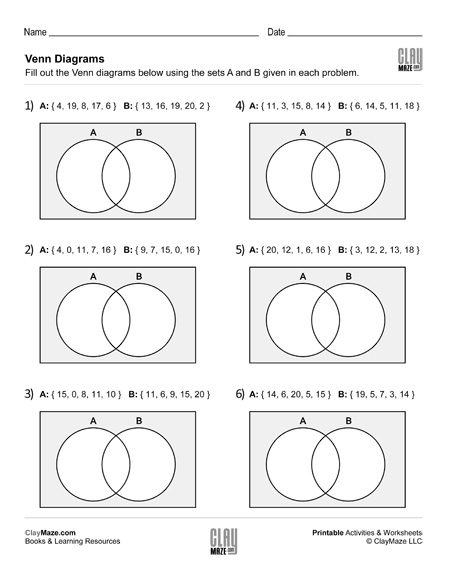Venn diagrams are a great exercise in learning how sets of numbers are related and how they differ. It also helps in data interpretation. For more explanation of what a Venn diagram is and to see...

## Venn Diagrams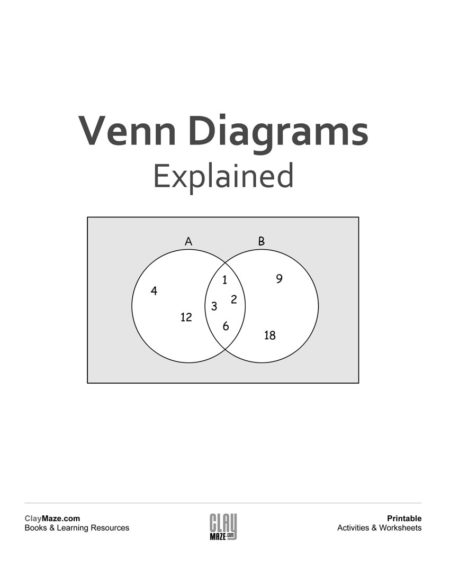A Venn diagram can be used to organize groups of things in a visual way to show how the groups are similar and how they are different. The similar objects of the groups are placed in the overlappin...

## Alphabetical Order – First and Last (Set 1)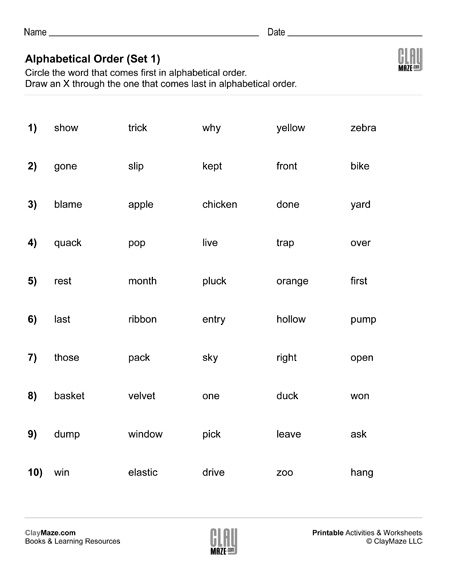In this worksheet, the student is asked to find the first and last words in alphabetical order for each group of words. There are five words in each group. This one is a fairly basic exercise in...

## Alphabetical Order – First and Last (Set 2)This is a starter worksheet on alphabetizing (the words in each group begin with a different letter). In each set of words, the student is asked to find the first word in alphabetical order and...

## Alphabetical Order – First and Last – Topic: General Science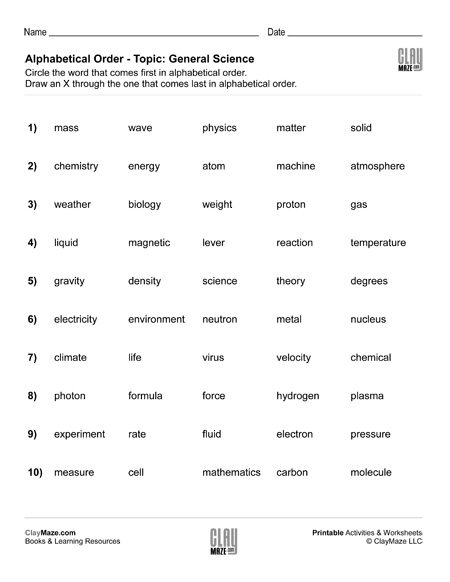The topic of this worksheet is general science and contains science vocabulary words. There are 5 words in each group and the student is asked to find the first and last words in alphabetical order...

## Alphabetical Order – First and Last – Topic: AstronomyThis worksheet is an exercise in alphabetizing. The student is asked to find the first and last words in alphabetical order for each group of words. Make sure the student reads each word...

## Alphabetical Order – First and Last – Topic: Math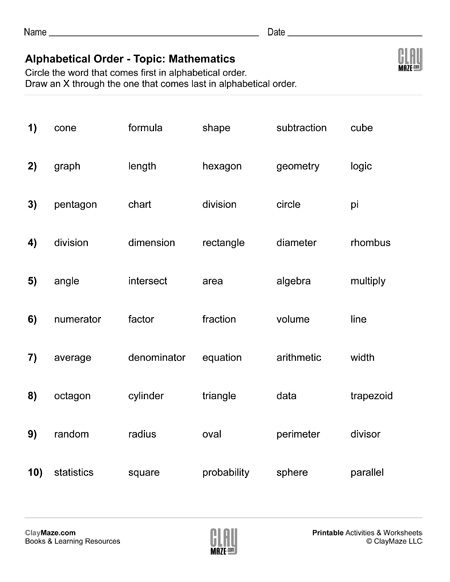This worksheet is for practice in sorting alphabetically and the topic of the worksheet is math, so the words in the lists are math terms. For each set of words, the student is to choose the first ...

## Alphabetical Order – First and Last – Topic: AnimalsThe topic of this worksheet is animals and is an exercise in sorting alphabetically. The student will need to find the first and last words alphabetically in each set. Some of the words in each...